Third graders will become more proficient mathematicians with these worksheets that cover the four basic math operations up to six exciting digits! They will be fascinated by new subjects such as graphing and data analysis, probability, fractions, decimals, and more. These subjects are covered in greater depth than many third grade worksheets and will challenge your students to be their mathematical best!Monthly Math Challenge Workbooks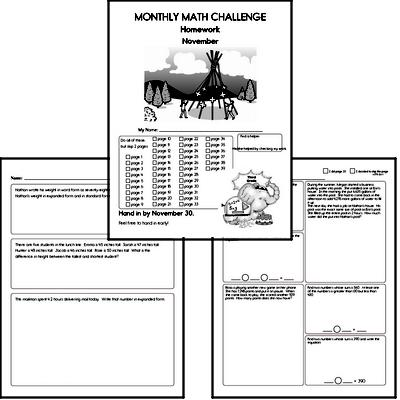Mental Math Workbooks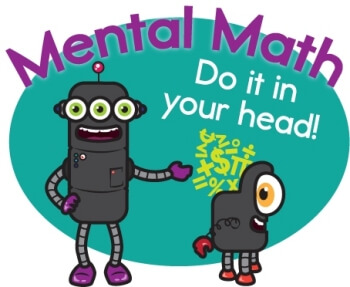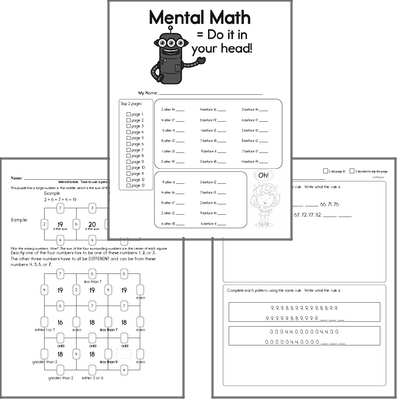Mental Math Workbooks

Third Grade Math Worksheets Activity Workbook - No Prep and Not Boring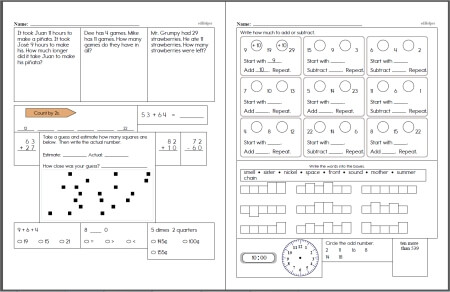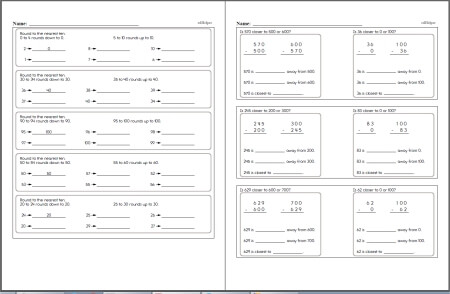Third Grade Math Book New Third Grade Math Workbook Created Each book contains word problems, mixed math, and other third grade review materials. Use it each week for daily practice in your classroom.

Daily Third Grade Math and Critical Thinking Practice

Third Grade Daily Homework and Practice Pages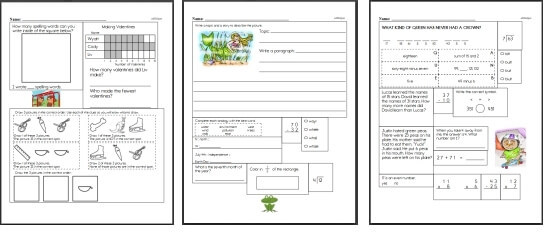Third Grade Daily Homework

Level 1: Easiest (First 2 months of school)
Level 2: Next 3 months of school
Level 3: Next 3 months of school
Level 4: Entire Grade (Final months of school)

Weekly Word Problems
Last week's third grade math word problems
This week's third grade math word problems
Next week's third grade math word problems
Daily Review: Customize math review skills with word problems, language arts, and science

Learning Multiplication Facts Book: You Pick Numbers to Focus On
Perfect for learning multiplication - especially the first page!

Learning Division Facts Book: You Pick Numbers to Focus On
Perfect for learning Division - especially the first page!

Runaway Math Puzzles
 Third grade runaway math puzzles Runaway Math Puzzles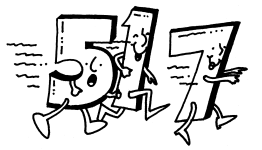Equation Unknowns Puzzle

Basic Operations
Subtraction
Multiplication
Division

Math Facts

Math Facts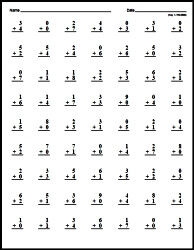Make Custom Quick Math Facts Printable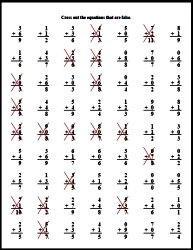Place Value

Place Value: Ones, Tens, and Hundreds
Write each number in standard form
Write each number in two other ways
Write the place and the value of the underlined digit
Fill in the missing number
Write each number in standard form (information given is mixed up)
Fill in the missing number (information given is mixed up)
Ones, Tens, and Hundreds Mixed Review

Place Value: Through Thousands, Ten Thousands, and Hundred Thousands
Write each number in standard form
Write each number in two other ways
Write the place and the value of the underlined digit
Fill in the missing number
Write each number in standard form (information given is mixed up)
Fill in the missing number (information given is mixed up)
Thousands, Ten Thousands, and Hundred Thousands Mixed Review

Rounding Whole Numbers
Round to the nearest ten
Round to the nearest hundred
Round to the nearest thousand
Write the 2 tens between each number
Write the 2 hundreds between each number
Write the 2 thousands between each number
Round to the place of the underlined digit (tens, hundreds, and thousands)
Fill in the missing digit (ones, tens, or hundreds digits blank)
Fill in the missing digit (tens, hundreds, or thousands digits blank)
Fill in the missing digit (hundreds, thousands, or ten-thousands digits blank)
Round to the nearest ten-thousand
Round to the nearest hundred-thousand
Round to the nearest million
Round to the place of the underlined digit (ten-thousands, hundred-thousands, and millions)
Round to the place of the underlined digit (ones through millions)
Rounding Whole Numbers Mixed Review

 Greater and Less Than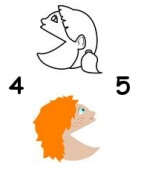Greater and Less Than

Money
Write in decimal form (money graphics)
Write in decimal form (sixty-five dollars and one cent)
Write in decimal form (4 one-dollar bills, 3 quarters, 1 dime)
Compare (money graphics)
Compare
Show amount using fewest number of coins (20 to 99 cents)
Show amount using fewest number of coins (20 to 99 cents; fill-ins have mixed order)
Show amount using fewest number of coins (\$0.20 to \$4.99)
Show amount using fewest number of coins (\$0.20 to \$19.99)
Make change using the given number of coins (20 to 99 cents)
Make change using the given number of coins (\$0.20 to \$4.99)
Make change using the given number of coins (\$0.20 to \$19.99)
Compare (\$1.59 ____ \$1.95)
Round to the nearest dollar
Round to the nearest ten dollars
Round to the place of the underlined digit
Problem solving
Money Mixed Review

2 digits with 3 numbers column addition
2-3 digits with 3 numbers column addition
2-5 digits with 3 numbers column addition
Fill in the missing numbers: 2 digits
Fill in the missing numbers: 2-3 digits
Fill in the missing numbers: 4-5 digits
Fill in the missing numbers: 2-6 digits
Math word puzzles
Math addition boxes: 3 x 3
Math addition boxes: 4 x 4

Number Bonds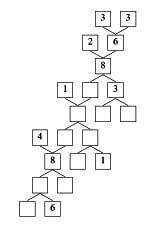Number Bonds

Super Silly Squares

Subtraction Squares

Subtraction
2 digits column subtraction
3 digits column subtraction
2-3 digits column subtraction
4 digits column subtraction
5-6 digits column subtraction
3-5 digits column subtraction
2 digits across subtraction
2-3 digits across subtraction
2-4 digits across subtraction
3-4 digits across subtraction
5-6 digits across subtraction
Column subtraction: start with 2 digits and increase by 1 digit in each row
Column subtraction: start with 2-3 digits and increase by 1 digits every 2 rows
Column subtraction: start with 2-3 digits and increase by 2 digits every 2 rows
Across subtraction with 3-5 numbers
Fill in the missing numbers: 2 digits
Fill in the missing numbers: 2-3 digits
Fill in the missing numbers: 4-5 digits
Fill in the missing numbers: 2-6 digits
Math word puzzles
Math addition boxes: 3 x 3
Math addition boxes: 4 x 4
Subtraction word problems
Subtraction Mixed Review

Slides, Turns, and Flips

Perimeter

Ordered Pairs and the Coordinate Plane

Graph and Analyze Data
Tally table
Frequency table
Make a bar graph from a tally table
Make a bar graph from a frequency table
Line plots
Stem-and-leaf plots
Vertical bar graphs
Vertical bar graphs (draw graph from table)
Horizontal bar graphs
Horizontal bar graphs (draw graph from table)
Ordered pairs: Write the ordered pair for each point
Ordered pairs: Find each ordered pair. Write the letter for the point named by the ordered pair.
Ordered pairs: Draw the ordered points on the grid. Label each point.
Line graphs
Line graphs (draw graph from table)
Graph and Analyze Data Mixed Review

Probability
Write whether each event is certain, likely, unlikely, or impossible
Probability problems (gives half of the answer)
Probability problems
Probability with spinners (gives half of the answer)
Probability with spinners
Probability Mixed Review

Introduction to Multiplication (Part 1)
Multiplication Concepts (with graphics of pictures)
Write the sum and product
Write the addition and multiplication fact
Write the sum and product (smaller graphics)
Write the addition and multiplication fact (smaller graphics)
Multiplication with Rows (dot graphics)
Multiply with 2
Multiply with 3
Multiply with 4
Multiply with 5
Multiply with 6
Mixed
Write the sum and then write the product
Multiply with 2
Multiply with 3
Multiply with 4
Multiply with 5
Multiply with 6
Multiply with 7
Multiply with 8
Multiply with 9
Multiply with 10
Mixed
Addition and Multiplication: Fill in the missing numbers
Multiply with 2
Multiply with 3
Multiply with 4
Multiply with 5
Multiply with 6
Multiply with 7
Multiply with 8
Multiply with 9
Multiply with 10
Mixed
Comparison
Introduction to Multiplication: Mixed Review
Introduction to Multiplication Mixed Review

Introduction to Multiplication (Part 2)
Basic Multiplication Worksheets
Multiply with 0 and 1
Multiply with 2
Multiply with 3
Multiply with 4
Multiply with 5
Multiply with 6
Multiply with 7
Multiply with 8
Multiply with 9
Multiply with 10
Mixed Basic Multiplication Worksheets (numbers 0 to 10)
Vertical multiplication
Horizontal multiplication
Vertical and horizontal multiplication
Fill in the missing number
Mixed Basic Multiplication Worksheets (numbers 2 to 10)
Vertical multiplication
Horizontal multiplication
Vertical and horizontal multiplication
Fill in the missing number
Mixed Basic Multiplication Worksheets with a smaller font (numbers 2 to 10)
Vertical multiplication
Horizontal multiplication
Multiplication Problem Solving
Multiplication problem solving
Multiplication Mixed Review
Multiplication Mixed Review

Introduction to Division
Division Concepts (with graphics of pictures)
Divide into groups (smaller numbers)
Divide into groups (larger numbers)
Divide into groups (mix)
Division Concepts with left over graphics
Divide into groups (smaller numbers)
Divide into groups (larger numbers)
Divide into groups (mix)
Division Facts (with graphics)
Write a division fact (smaller numbers)
Write a division fact (larger numbers)
Write a division fact (mix)
Multiplication and Division
Fill in the quotient
Fill in the missing number
Fill in the missing number
Division Problem Solving
Division problem solving
Division Mixed Review
Introduction to Division Mixed Review

Division Facts Through 10
Multiplication and Division: Fill in the missing numbers
Multiplication and division with 2
Multiplication and division with 3
Multiplication and division with 4
Multiplication and division with 5
Multiplication and division with 6
Multiplication and division with 7
Multiplication and division with 8
Multiplication and division with 9
Multiplication and division with 10
Multiplication and division (mix)
Division Facts
Divide by 2
Divide by 3
Divide by 4
Divide by 5
Divide by 6
Divide by 7
Divide by 8
Divide by 9
Divide by 10
Division (mix)
Division Facts
Division by 2 to 10
Division by 2 to 10
Fill in the missing number
Division Problem Solving
Division problem solving
Division Mixed Review
Division Facts Mixed Review

Understand Fractions
Write a fraction to show how much of the shape is shaded
Write a fraction to show how much of the shape is not shaded
Draw a picture to show the fraction
Same numerators
Same denominators
Either the same numerators or the same denominators
Fraction Comparison (with graphics, but not shaded in)
Same numerators
Same denominators
Fill in the missing number to make two equivalent fractions
Fill in one number: Larger graphics with fractions shown
Fill in one number: Smaller graphics with only boxes
Fill in two number: Larger graphics with fractions shown
Fill in two number: Smaller graphics with only boxes
Mixed Numbers and Improper Fractions
Multiple choice: Circle the correct improper fraction for the parts that are shaded
Multiple choice: Circle the correct mixed number for the parts that are shaded
Write an improper fraction for the parts that are shaded
Write a mixed number for the parts that are shaded
Problem Solving
Single step
Two steps
Mixed Review
Understand Fractions Mixed Review

Fraction comparison
Order fractions from least to greatest
Order fractions from greatest to least
Rewrite each improper fraction as a mixed number
Add fractions: Find the value of y
Subtract fractions
Subtract fractions: Find the value of y
Add and subtract fractions: Find the value of y
Add and Subtract Like Fractions Mixed Review

Understanding Decimals
Tenths
Write each as a decimal (given: graphics, fractions, and word form)
Given graphics, write the decimal (decimals greater than 1)
Write each as a decimal (decimals greater than 1)
Write each as a fraction

Tenths and Hundredths
Hundredths: Write each as a fraction and decimal
Hundredths: Write each as a decimal
Hundredths: Write each as a fraction
Tenths and hundredths: Write each as a decimal
Tenths and hundredths: Write each as a fraction
Hundredths: Write each as a decimal (graphics)
Tenths and hundredths greater than 1: Write each as a decimal
Tenths and hundredths greater than 1: Write each as a fraction
Tenths and hundredths: Write each as a decimal (mix: less than and greater than 1 decimals)
Tenths and hundredths: Write each as a fraction (mix: less than and greater than 1 decimals)
Tenths and hundredths: Compare decimals
Tenths and hundredths: Order decimals
Order decimals (from 6 to 8 numbers each)
Compare decimals and fractions
Order decimals and fractions
Order decimals and fractions (from 7 to 11 numbers each)
Compare decimals and fractions (with numbers greater than 1)
Order decimals and fractions (with numbers greater than 1)
Tenths and Hundredths Mixed Review

Thousandths
Thousandths: Write as a decimal
Thousandths: Write as a fraction
Thousandths: Write as a decimal (decimals greater than 1)
Thousandths: Write as a fraction (decimals greater than 1)

Tenths, Hundredths, and Thousandths
Write as a decimal
Write as a fraction
Compare decimals
Ordering decimals
Compare decimals (including fractions)
Ordering decimals (including fractions)

Rounding Decimals
Rounding: Round to the nearest tenth
Rounding: Round to the nearest hundredth
Rounding: Round to the place of the underlined digit

Review
Mixed Review

Column addition of 2 numbers (up to tenths)
Column addition of 2 numbers (up to hundredths)
Column addition of 2 numbers (up to thousandths)
Money column addition (up to \$99.99)
Money column addition (up to \$99,999.99)
Across addition of 2 numbers (up to hundredths)
Across addition of 2 numbers (up to thousandths)

Subtraction
Column subtraction of 2 numbers (up to tenths)
Column subtraction of 2 numbers (up to hundredths)
Column subtraction of 2 numbers (up to thousandths)
Money column subtraction (up to \$99.99)
Money column subtraction (up to \$99,999.99)
Across subtraction of 2 numbers (up to hundredths)
Across subtraction of 2 numbers (up to thousandths)

Column addition and subtraction of 2 numbers (up to tenths)
Column addition and subtraction of 2 numbers (up to hundredths)
Column addition and subtraction of 2 numbers (up to thousandths)
Money column addition and subtraction (up to \$99.99)
Money column addition and subtraction (up to \$99,999.99)
Across addition and subtraction of 2 numbers (up to hundredths)
Across addition and subtraction of 2 numbers (up to thousandths)

Part 2
Order of Operations (no parenthesis)
Order of Operations
Order of Operations (one missing number)
Order of Operations (one missing operation)
Order of Operations (fill in all numbers)
Order of Operations (fill in all operations)
Fill in the missing numbers (up to hundredths)
Fill in the missing numbers (up to thousandths)
Math box
Decimal addition and subtraction word problems
Addition and subtraction of decimals: Mixed Review

Division by One-Digit Numbers
Column Division with Extra Space To Solve Problems - Division by One-Digit Numbers
2 digits by 1 digit column division (no remainders)
2-3 digits by 1 digit column division (no remainders)
4-5 digits by 1 digit column division (no remainders)
5-7 digits by 1 digit column division (no remainders)
2 digits by 1 digit column division
2-3 digits by 1 digit column division
4-5 digits by 1 digit column division
5-7 digits by 1 digit column division
2 digits (and increasing 1 digit every 2 rows) by 1 digit column division
2 digits (and increasing 1 digit every row) by 1 digit column division

Column Division (more problems with less space) - Division by One-Digit Numbers
2 digits by 1 digit column division (no remainders)
2-3 digits by 1 digit column division (no remainders)
4-5 digits by 1 digit column division (no remainders)
5-7 digits by 1 digit column division (no remainders)
2 digits by 1 digit column division
2-3 digits by 1 digit column division
4-5 digits by 1 digit column division
5-7 digits by 1 digit column division
2 digits (and increasing 1 digit every 2 rows) by 1 digit column division
2 digits (and increasing 1 digit every row) by 1 digit column division

Part 2 - Division by One-Digit Numbers
Divisibility: complete the table
2 digits by 1 digit across division
2-3 digits by 1 digit across division
4-5 digits by 1 digit across division
2 digits (and increase by 1 digit every row) by 1 digit across division
Fill in the missing numbers: 2 digits by 1 digit
Fill in the missing numbers: 3 digits by 1 digit
Fill in the missing numbers: 4 digits by 1 digit
Division Word Problems
Division Word Problems
Solve equations: 2 digits by 1 digit
Order of operations: 2-3 digits by 1 digit (no parenthesis)
Order of operations: 2-3 digits by 1 digit
Order of operations: 2-4 digits by 1 digit
One-Digit Numbers Division Mixed Review

Multiplication
Column Multiplication with Extra Space To Solve Problems
1-2 digits by 1 digit column multiplication
2 digits (and increasing 1 digit every 2 rows) by 1 digit column multiplication
2 digits (and increasing 1 digit every row) by 1 digit column multiplication
Multiples of 10 multiplication (2-5 digits by 1 digit)
2 digits by 2 digits column multiplication
2-3 digits by 2 digits column multiplication
4-5 digits by 2 digits column multiplication
2 digits (and increasing 1 digit every 2 rows) by 2 digit column multiplication
2 digits (and increasing 1 digit every row) by 2 digit column multiplication

Column Multiplication (more problems with less space)
1-2 digits by 1 digit column multiplication
2 digits (and increasing 1 digit every 2 rows) by 1 digit column multiplication
2 digits (and increasing 1 digit every row) by 1 digit column multiplication
Multiples of 10 multiplication (2-5 digits by 1 digit)
2 digits by 2 digits column multiplication
2-3 digits by 2 digits column multiplication
4-5 digits by 2 digits column multiplication
2 digits (and increasing 1 digit every 2 rows) by 2 digit column multiplication
2 digits (and increasing 1 digit every row) by 2 digit column multiplication

Part 2
1-2 digits by 1 digit across multiplication
3-4 digits by 1 digit across multiplication
1-2 digits by 2 digits across multiplication
Across multiplication with 3-5 numbers
Fill in the missing numbers: 1-2 digits
Fill in the missing numbers: 2 digits
Fill in the missing numbers: 2-3 digits
Math multiplication boxes: 3 x 3 (numbers 1-9)
Math multiplication boxes: 4 x 4 (numbers 1-9)
Math multiplication boxes: 3 x 3 (numbers 4-19)
Multiplication word problems (basic)
Multiplication word problems
Multiplication word problems
Multiplication Mixed Review

Test Prep - Concepts
from the test prep section, requires registration
Numeration
Number Concepts
Properties
Fractions and Decimals
Review of Number Concepts

Test Prep - Computation
from the test prep section, requires registration
Subtraction
Multiplication
Division
Review of Computation

Test Prep - Applications
from the test prep section, requires registration
Geometry
Measurement
Problem Solving - Word Problems!
Problem Solving Applications (Charts, etc)

Have a suggestion or would like to leave feedback?